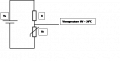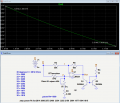# Thermistor - Voltage Circuit.

Thread Starter

#### NMC

Joined Nov 16, 2018
13
Hi All,

I am looking to design a circuit using an Omega4404 thermistor which at 20 degree's outputs 0v.
I want to draw a graph of corresponding voltages for 20-30 degrees.

What type of circuit do I need to design?

Thanks
N

#### ericgibbs

Joined Jan 29, 2010
12,269
hi NMC,
Do you have a datasheet for that 4404 sensor.?
E

#### AlbertHall

Joined Jun 4, 2014
11,139

#### Alec_t

Joined Sep 17, 2013
11,984
Jeepers! That 44004 is expensive! There are MUCH cheaper thermistors available and which may do the job, unless you are constrained to have that particular resistance (2252Ω @ 25C).
What tolerance is acceptable for your purpose?
What is the purpose?

Last edited:

#### MrChips

Joined Oct 2, 2009
23,090
Thermistors, in general, are non-linear resistors with negative temperature coefficients (NTC), i.e. resistance goes down as temperature goes up. The simplest way to get a linear response is to get the data into a computer and do the data to temperature conversion mathematically.

If you want 0V output at any specific temperature, you can use a difference amplifier to subtract any DC offset voltage or use a bridge circuit.

Personally, I prefer to convert the thermistor resistance to a frequency and get a microcontroller (MCU) to read the frequency. Without knowing your specific application it is difficult to suggest any such options.

#### sghioto

Joined Dec 31, 2017
2,272
Fahrenheit or celcius? Did you mean Omega 44004?
SG

Thread Starter

#### NMC

Joined Nov 16, 2018
13
Hi all,

Thanks for the replies.
Apologies it is an Omega 44004.

I want to design a circuit that at 20 degrees Celsius the output voltage is 0V.
I then need to determine the voltage for temperatures 21-30 degrees Celsius which have given resistances as below:

20 degrees C = 2814 Ohms
21 = 2690
22 = 2572
23 = 2460
24 = 2354
25 = 2252
26 = 2156
27 = 2064
28 = 1977
29 = 1894
30 = 1815

Below is a circuit which I tried to simulate -#### KeepItSimpleStupid

Joined Mar 4, 2014
4,566
Look at the LTC 2983 / 2084 and 2986 IC's. It's a pricey IC.

#### crutschow

Joined Mar 14, 2008
26,789
Here's the bridge circuit with an op amp differential to single-ended output (LTspice simulation below).

Q1 and Q2 generate a current load on the output to help pull the output close to zero, which single-supply op amps don't generally do.
If you have a plus and minus supply for the amp, then those aren't needed.

The circuit gain is 2 as compared to the voltage across the same bridge connected to ground.

Pot U2 is for offset adjustment.

As you can see, the output voltage goes from ≈0V to ≈1V for a temp change of 20 to 30°C.Last edited:
•sghioto
Thread Starter

#### NMC

Joined Nov 16, 2018
13
Hi All,

Thanks for the replies again.
I'm still struggling to design a circuit for my thermistor-voltage circuit.
Do I use a simple bridge circuit or do I need to use some sort of op-amp?

N.

#### crutschow

Joined Mar 14, 2008
26,789
Do I use a simple bridge circuit or do I need to use some sort of op-amp?
So you don't understand the previous posts here?

#### joeyd999

Joined Jun 6, 2011
4,477
Personally, I prefer to convert the thermistor resistance to a frequency and get a microcontroller (MCU) to read the frequency.
Why?

#### MrChips

Joined Oct 2, 2009
23,090
Because an MCU is better at counting pulses than measuring analog voltages.

#### joeyd999

Joined Jun 6, 2011
4,477
Because an MCU is better at counting pulses than measuring analog voltages.
Seriously: I would love to see your calibrationless T-to-F solution that gives milli°C resolution and centi°C accuracy with only one external component besides the MCU and thermistor.

Edit: I've discussed my solution.

#### MrChips

Joined Oct 2, 2009
23,090
Seriously, no one is talking about calibrationless temperature measurement. The thermistor is non-linear and some form of transformation is required. Thermistors are interchangeable once the system is calibrated.

My solution consists of thermistor, capacitor, and MCU.

#### joeyd999

Joined Jun 6, 2011
4,477
My solution consists of thermistor, capacitor, and MCU.
And calibration. How's the stability against temperature and Vdd/reference voltage changes?

#### crutschow

Joined Mar 14, 2008
26,789
My solution consists of thermistor, capacitor, and MCU.
How does that accurately convert the thermistor resistance to a frequency?#### MrChips

Joined Oct 2, 2009
23,090
How does that accurately convert the thermistor resistance to a frequency?I charge the capacitor then allow the capacitor to discharge through the resistor. I do this many times and then count how many times I can do this in a certain time period.

#### crutschow

Joined Mar 14, 2008
26,789
I charge the capacitor then allow the capacitor to discharge through the resistor. I do this many times and then count how many times I can do this in a certain time period.
And you really think that's more accurate than using the processors A/D converter to measure an analog voltage, such as from the bridge circuit?

Similar threads